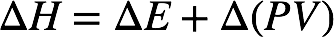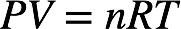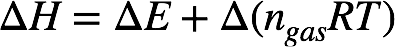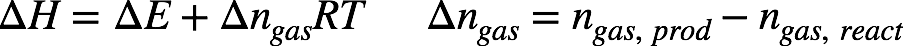# Problem: The heat of combustion of liquid octane (C8H18) to carbon dioxide and liquid water at 298 K is -1303 kJ/mol. Find ΔE for this reaction.

###### FREE Expert Solution

The change in enthalpy ΔH and change in internal energy ΔE is related by the following equation:From the ideal gas law, we have:Plugging this into the first equation:where ngas = moles of gas in the reaction, R = universal gas constant, T = temperature. At a given temperature:89% (354 ratings)###### Problem Details

The heat of combustion of liquid octane (C8H18) to carbon dioxide and liquid water at 298 K is -1303 kJ/mol. Find ΔE for this reaction.